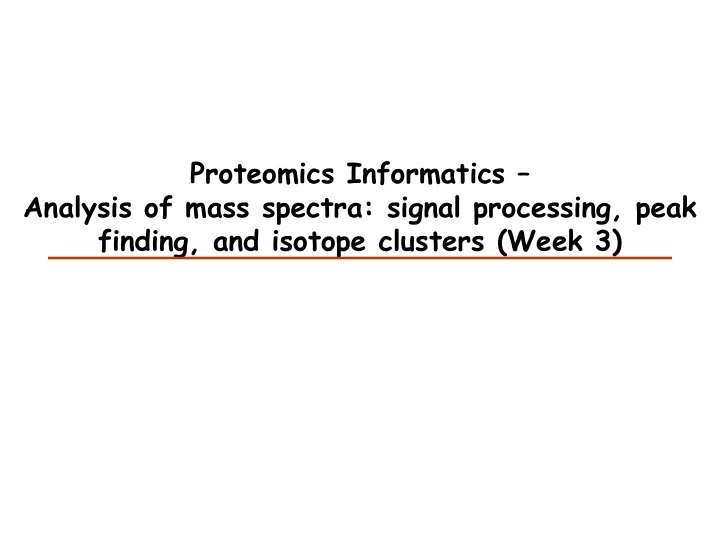# Proteomics Informatics Analysis of mass spectra: signal processing, - PowerPoint PPT Presentation

## Proteomics Informatics Analysis of mass spectra: signal processing, peak finding, and isotope clusters (Week 3) Charge-State Distributions MALDI ESI 1+ 2+ intensity intensity 3+ Peptide 4+ 1+ 2+ mass/charge mass/charge m M nH

1. Proteomics Informatics – Analysis of mass spectra: signal processing, peak finding, and isotope clusters (Week 3)

2. Charge-State Distributions MALDI ESI 1+ 2+ intensity intensity 3+ Peptide 4+ 1+ 2+ mass/charge mass/charge m M nH + = M - molecular mass z n n - number of charges H – mass of a proton MALDI ESI 2+ 27+ intensity intensity 1+ 3+ 31+ Protein 4+ 5+ mass/charge mass/charge

3. Charge-State m M nH + = M - molecular mass z n n - number of charges H – mass of a proton Example: peptide of mass 898 carrying 1 H + = (898 + 1) / 1 = 899 m/z carrying 2 H + = (898 + 2) / 2 = 450 m/z carrying 3 H + = (898 + 3) / 3 = 300.3 m/z

4. Isotope Distributions m = 1035 Da m= 1878 Da m = 2234 Da 12 C 14 N Intensity +1Da 16 O 1 H 32 S +2Da +3Da m/z m/z m/z 0.015% 2 H 1.11% 13 C 0.366% 15 N 0.038% 17 O, 0.200% 18 O, 0.75% 33 S, 4.21% 34 S, 0.02% 36 S Only 12 C and 13 C: p=0.0111 n is the number of C in the peptide m is the number of 13 C in the peptide T m is the relative intensity of the peptide m 13 C

5. Isotope distributions Intensity ratio Intensity ratio Peptide mass Peptide mass GFP 29kDa monoisotopic mass m/z

6. Resolution M = resolving power R = ∆ M Resolution = minimum peak separation, ∆ M, which allows to distinguish two ion species ∆ M = full width at half maximum (FWHM) 500 Relative Intensity 50 % I I I I I 499.5 500.0 500.5 501.5 502.0 m/z Resolution = M/ ∆ M = 500/0.5 = 1000

7. Resolution

8. Resolution M = resolving power R = ∆ M • What resolution do we need to differentiate a 1600 Da peptide that carries either an acetylation (+ 42.0100) or trimethylation (42.0464 )? • R = 1600/0.0364 = 43,956

9. Isotope Clusters and Charge State 1 1+ Intensity 1 1 m/z 0.5 2+ Intensity 0.5 0.5 m/z 0.33 3+ Intensity 0.33 0.33 m/z

10. Isotope Clusters and Charge State Possible to Determine Charge? Intensity Yes m/z Yes Maybe No

11. What is the Charge State? 713.3225 432.8990 713.8239 433.2330 714.3251 433.5671 433.9014 714.8263 ∆ between the ∆ between the isotopes is 0.5 Da isotopes is 0.33 Da

12. Noise

13. Smoothing

14. Smoothing

15. Adaptive Background Correction (Unsharp masking) = + k l w d ∑ = I l d w I k ' ( , , ) ( ) + 2 w 1 = − k l w Original Unsharp masking

17. Smoothing and Adaptive Background Correction

18. Peak Finding Intensity Find maxima of = + k l w ∑ = S l I k ( ) ( ) m/z = − k l w The centroid m/z of a peak = + k l w m ∑ ⋅ k I ( k ) ( ) z = − k l w = + k l w ∑ I ( k ) = − k l w

19. Peak Finding Intensity The signal in a peak can be m/z estimated with the RMSD ( I ( k ) I ) − < > ∑ 2 | k l | w / − < 2 w / 2 and the signal-to-noise ratio of a peak can be estimated by dividing the signal with the RMSD of the background

20. Estimating peptide quantity Peak height Peak height Curve fitting Curve fitting Intensity Peak area m/z

21. Time dimension Intensity m/z Time Time m/z

22. Sampling Intensity Retention Time

23. Sampling 140 3 points 120 100 80 60 5% 40 20 0 0.8 0.85 0.9 0.95 1 30 3 points 25 20 5% 15 10 5 0 0.8 0.85 0.9 0.95 1 Acquisition time = 0.05 σ

24. Sampling 1.1 1 Thresholds (90%) 0.9 0.8 0.7 0.6 0.5 1 2 3 4 5 6 7 8 9 10 # of points

25. What is the best way to estimate quantity? Peak height - resistant to interference - poor statistics Peak area - better statistics - more sensitive to interference Curve fitting - better statistics - needs to know the peak shape - slow

26. Web Tool http://10.193.36.101/plot-filter-cgi/plot_filter.pl or http://10.193.36.219/plot-filter-cgi/plot_filter.pl

27. Web Tool http://10.193.36.101/plot-filter-cgi/plot_filter.pl or http://10.193.36.219/plot-filter-cgi/plot_filter.pl

28. Proteomics Informatics – Analysis of mass spectra: signal processing, peak finding, and isotope clusters (Week 3)

More recommend